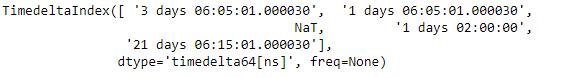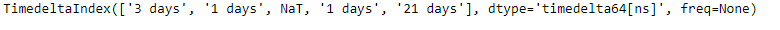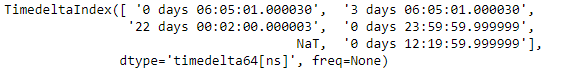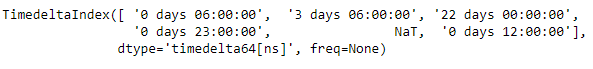Related Articles
Python | Pandas TimedeltaIndex.floor
• Last Updated : 28 Dec, 2018

Python is a great language for doing data analysis, primarily because of the fantastic ecosystem of data-centric python packages. Pandas is one of those packages and makes importing and analyzing data much easier.

Pandas` TimedeltaIndex.floor()` function floor all the values in the given TimedeltaIndex object to the specified frequency.

Syntax : TimedeltaIndex.floor(freq)

Parameters :
freq : freq string/object

Return : index of same type

Example #1: Use `TimedeltaIndex.floor()` function to floor all the values in the given TimedeltaIndex object to daily frequency.

 `# importing pandas as pd``import` `pandas as pd`` ` `# Create the TimedeltaIndex object``tidx ``=` `pd.TimedeltaIndex(data ``=``[``'3 days 06:05:01.000030'``, ``'1 days 06:05:01.000030'``,``                               ``None``, ``'1 days 02:00:00'``, ``'21 days 06:15:01.000030'``])`` ` `# Print the TimedeltaIndex object``print``(tidx)`

Output :Now we will use the `TimedeltaIndex.floor()` function to floor all the values.

 `# floor the values to daily frequency``tidx.floor(freq ``=``'D'``)`

Output :As we can see in the output, the `TimedeltaIndex.floor()` function has floored all the values to the daily frequency.

Example #2: Use `TimedeltaIndex.floor()` function to floor all the values in the given TimedeltaIndex object to hourly frequency.

 `# importing pandas as pd``import` `pandas as pd`` ` `# Create the TimedeltaIndex object``tidx ``=` `pd.TimedeltaIndex(data ``=``[``'06:05:01.000030'``, ``'3 days 06:05:01.000030'``,``                                ``'22 day 2 min 3us 10ns'``, ``'+23:59:59.999999'``,``                                                  ``None``, ``'+12:19:59.999999'``])`` ` `# Print the TimedeltaIndex object``print``(tidx)`

Output :Now we will use the `TimedeltaIndex.floor()` function to floor all the values.

 `# floor the values to hourly frequency``tidx.floor(``'H'``)`

Output :As we can see in the output, the `TimedeltaIndex.floor()` function has floored all the values to the hourly frequency.

Attention geek! Strengthen your foundations with the Python Programming Foundation Course and learn the basics.

To begin with, your interview preparations Enhance your Data Structures concepts with the Python DS Course. And to begin with your Machine Learning Journey, join the Machine Learning – Basic Level Course

My Personal Notes arrow_drop_up